# How to Calculate Depreciation Expense

Depreciation is an important concept to learn as it applies with all the fixed assets in an organization. Under the category of fixed assets, there are buildings, equipments and machinery, furniture, vehicles, etc. When these items are used, the initial value of these assets reduces or depreciates over time. Therefore, in organizational point of view, the reducing amount needs to be included in accounts as an expense. In this article, various methods used in accounting for depreciation in the financial statements are discussed.

## Methods of Calculating Depreciation Expense

There are two methods that are most frequently used in accounting to calculate depreciation expense as indicated below:

• Straight Line Method
• Reducing Balance Method

### Straight Line Method of depreciation

In Straight Line method, the cost is depreciated equally among the useful life of the fixed assets. The following formula for calculating depreciation can be used.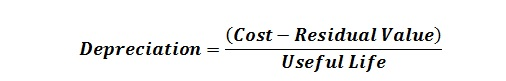Where,

• Cost represents the initial price or value of the fixed asset.
• Residual Value refers to the estimated scrap value at the end of the useful life of a fixed asset.
• Useful Life is the estimated lifetime of the fixed asset that can be used until the time of the disposal. It can be calculated in the units of years.

For example, the purchase cost of the machine is \$60,000 and the estimated residual value is \$10,000, and the useful life of the asset is five years. The depreciation is calculated based on the straight line method and the following formula for calculating depreciation can be used.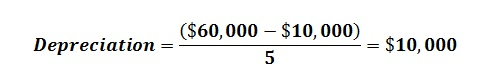The depreciation calculation can be recorded in the balance sheet in the following manner: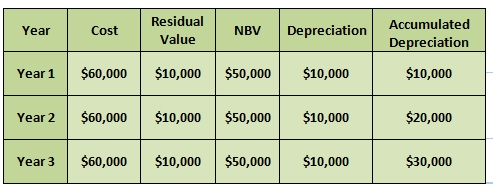Double entry for the depreciation can be illustrated as follows :

###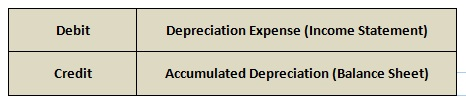Reducing Balance Method of Depreciation

The depreciation amount is calculated by way of reducing the cost of the asset over time. The depreciation value is calculated using the following formula: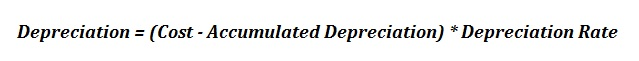By reducing the accumulated depreciation from the cost of the fixed asset, the Net Book Value (NBV) can be generated. According to this method, depreciation amount is calculated based on the NBV.

For an example, Company X bought a fixed asset with the cost of \$2,000 and its residual value is \$500. The useful life of the asset is three years and the rate of depreciation is 50%. Here, the depreciation amount is calculated based on the reducing balance method.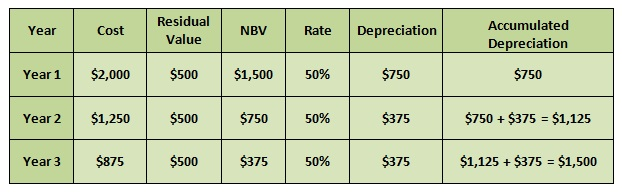Reducing Balance Method is suitable for assets which have a higher capacity at the initial stages of life such as computer equipments, machines, etc. It ensures to charge a higher depreciation at the earlier stages of life.